## Selina Concise Mathematics Class 7 ICSE Solutions Chapter 20 Mensuration

Selina Publishers Concise Mathematics Class 7 ICSE Solutions Chapter 20 Mensuration

APlusTopper.com provides step by step solutions for Selina Concise ICSE Solutions for Class 7 Mathematics. You can download the Selina Concise Mathematics ICSE Solutions for Class 7 with Free PDF download option. Selina Publishers Concise Mathematics for Class 7 ICSE Solutions all questions are solved and explained by expert mathematic teachers as per ICSE board guidelines.

### Mensuration Exercise 20A – Selina Concise Mathematics Class 7 ICSE Solutions

Question 1.
The length and the breadth of a rectangular plot are 135 m and 65 m. Find, its perimeter and the cost of fencing it at the rate of ₹60 per m.
Solution:
Given :
Length (l) = 135 mPerimeter = 2 (l + b)
= 2(135 + 65)
= 2(200) = 400 m
∴Perimeter of rectangular plot is = 400 m
Cost of fencing per m = ₹60
∴Cost of fencing 400 m = ₹60 x 400 m = ₹24000

Question 2.
The length and breadth of a rectangular field are in the ratio 7 : 4. If its perimeter is 440 m, find its length and breadth. Also, find the cost of fencing it @ ₹150 per m.
Solution:
Given : Perimeter = 440 m
Let the length of rectangular field = lx and breadth = 4x
2(l + b) = Perimeter
2(7x + 4x) = 440 m
2(11x) = 440 m
22x = 440 m
x = $$\frac { 440 }{ 22 }$$
x = 11 m
∴Length = 7x = 7 x 11 = 77 m
Breadth = Ax = 4 x 11 = 44 m
Cost of fencing per m = ₹150
Cost of fencing 440 m = ₹150 x 440 = ₹66,000

Question 3.
The length of a rectangular field is 30 m and its diagonal is 34 m. Find the breadth of the field and its perimeter.
Solution: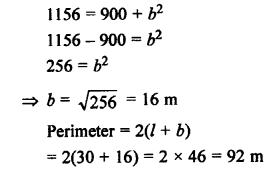Question 4.
The diagonal of a square is 12$$\sqrt { 2 }$$ cm. Find its perimeter.
Solution:
Diagonal of square = Its side x $$\sqrt { 2 }$$
Side $$\sqrt { 2 }$$ = $$\sqrt { 2 }$$ $$\sqrt { 2 }$$
i.e. side = 12 cm
Perimeter of a square = 4 x Side
= 4 x 12 = 48 cm

Question 5.
Find the perimeter of a rectangle whose length = 22.5 m and breadth = 16 dm.
Solution:
Length = 22.5 m
Breadth = 16 dm = 1.6 m
Perimeter of rectangle = 2(l + b)
– 2(22.5 + 1.6)
– 2(24.1) = 48.2 m

Question 6.
Find the perimeter of a rectangle with length = 24 cm and diagonal = 25 cm
Solution:
Length of a rectangle (l) = 24 cm Diagonal = 25 cm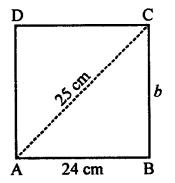Let breadth of the rectangle = b m
Applying Pythagoras Theorem in triangle ABC,
We get, (AC)2 = (AB)2 + (BC)2
(25)= (24)2 + (b)2
625 = 576 + (b)2
625 – 576 = b2
49 = A2
$$\sqrt { 7 x 7 }$$ =b
∴b = 7 cm
Now, perimeter of the rectangle
= 2(1 + b)
= 2(24 + 7)
= 2(31)
= 62 cm

Question 7.
The length and breadth of rectangular piece of land are in the ratio of 5 : 3. If the total cost of fencing it at the rate of ₹48 per metre is ₹19,200, find its length and breadth.
Solution:
Ratio in length and breadth of a rectangular piece of land = 5:3
Cost of fencing =₹ 19,200
and rate = ₹48 per m
∴Perimeter = $$\frac { 19200 }{ 48 }$$= 400 m 48
Let length = 5x.
∴Perimeter = 2(l + b)
400 = 2(5x + 3x)
400 = 2 x 8x= 16x
∴16x = 400
⇒ x = $$\frac { 400 }{ 16 }$$ = 25
∴Length of the land = 5x= 5 x 25 = 125 m and breadth = 3x = 3 x 25 = 75 m

Question 8.
A wire is in the shape of square of side 20 cm. If the wire is bent into a rectangle of length 24 cm, find its breadth.
Solution:
Side of square = 20 cm
Perimeter of square = 4 x 20 = 80 cm
Or perimeter of rectangle = 80 cm
Length of a rectangle = 24 cm
∴ Perimeter of a rectangle = 2(l + b)
b = $$\frac { 80 }{ 2 }$$ – 24
b = 40 – 24 = 16 m

Question 9.
If P = perimeter of a rectangle, l= its length and b = its breadth find :
(i) P, if l = 38 cm and b = 27 cm
(ii) b, if P = 88 cm and l = 24 cm
(iii) l, if P = 96 m and b = 28 m
Solution:Question 10.
The cost of fencing a square field at the rate of
Cost of fencing 440 m = ₹150 x 440 = ₹75 per meter is
Cost of fencing 440 m = ₹150 x 440 = ₹67,500. Find the perimeter and the side of the square field.
Solution: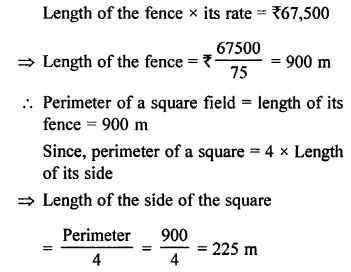Question 11.
The length and the breadth of a rectangle are 36 cm and 28 cm. If its perimeter is equal to the perimeter of a square, find the side of the square.
Solution: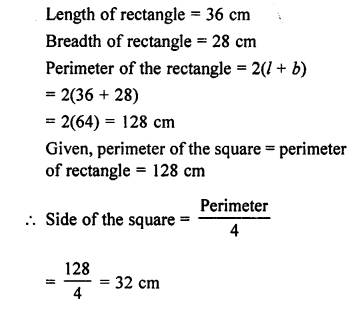Question 12.
The radius of a circle is 21 cm. Find the circumference (Take π = 3 $$\frac { 1 }{ 7 }$$ ).
Solution:Question 13.
The circumference of a circle is 440 cm. Find its radius and diameter. (Take π = $$\frac { 22 }{ 7 }$$
Solution: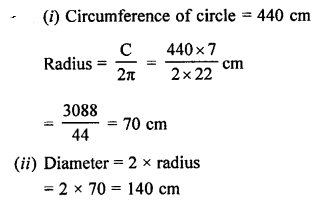Question 14.
The diameter of a circular field is 56 m. Find its circumference and cost of fencing it at the rate of ₹80 per m. (Take n = $$\frac { 22 }{ 7 }$$)
Solution: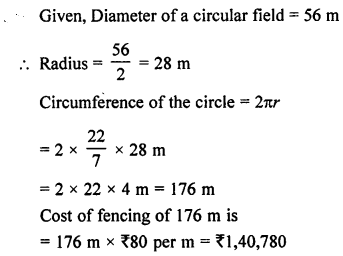Question 15.
The radii of two circles are 20 cm and 13 cm. Find the difference between their circumferences. (Take π = $$\frac { 22 }{ 7 }$$)
Solution: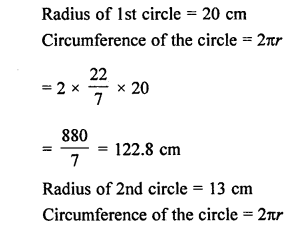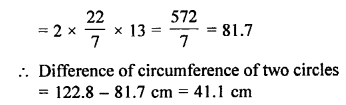Question 16.
The diameter of a circle is 42 cm, find its perimeter. If the perimeter of the circle is doubled, what will be the radius of the new circle. (Take π = $$\frac { 22 }{ 7 }$$ )
Solution: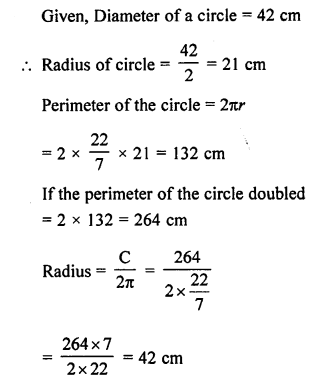Question 17.
The perimeter of a square and the circumference of a circle are equal. If the length of each side of the square is 22 cm, find:
(i) perimeter of the square.
(ii) circumference of the circle.
Solution: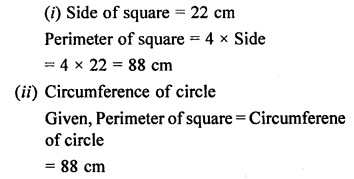Question 18.
Find the radius of the circle whose circumference is equal to the sum of the circumferences of the circles having radii 15 cm and 8 cm.
Solution:Question 19.
Find the diameter of a circle whose circumference is equal to the sum of circumference of circles with radii 10 cm, 12 cm and 18 cm.
Solution: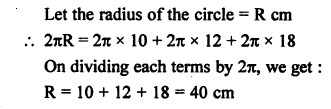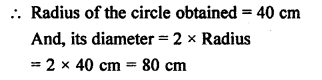Question 20.
The circumference of a circle is eigth time the circumference of the circle with radius 12 cm. Find its diameter.
Solution:Question 21.
The radii of two circles are in the ratio 3 : 5, find the ratio between their circumferences.
Solution:Question 22.
The circumferences of two circles are in the ratio 5 : 7, find the ratio between their radii.
Solution: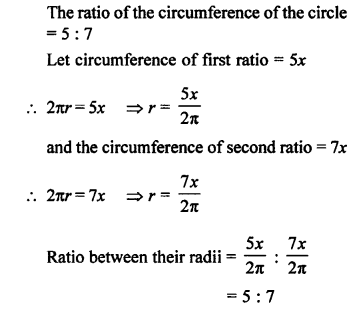Question 23.
The perimeters of two squares are in the ratio 8:15, find the ratio between the lengths of their sides.
Solution: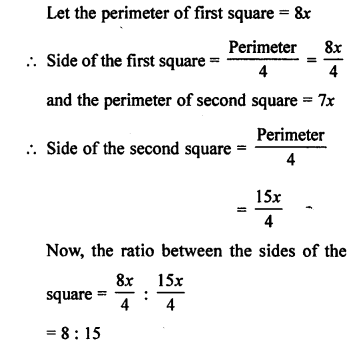Question 24.
The lengths of the sides of two squares are in the ratio 8:15, find the ratio between their perimeters.
Solution:
Let the side of first square = 8x
∴Perimeter of first square = 4 x Side = 4 x 8x = 32 x
and the side of second squares = 15x
∴Perimeter of second square = 4 x Side = 4 x 15s = 60s
Now, the ratio between their perimeter = 32x: 60x= 8: 15

Question 25.
Each side of a square is 44 cm. Find its perimeter. If this perimeter is equal to the circumference of a circle, find the radius of the circle.
Solution: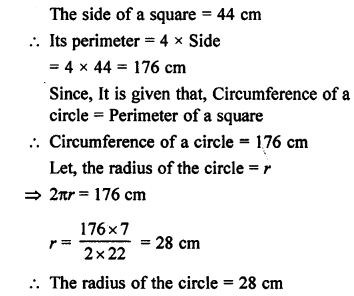### Mensuration Exercise 20B – Selina Concise Mathematics Class 7 ICSE Solutions

Question 1.
Find the area of a rectangle whose length and breadth are 25 cm and 16 cm.
Solution:Question 2.
The diagonal of a rectangular board is 1 m and its length is 96 cm. Find the area of the board.
Solution:Question 3.
The sides of a rectangular park are in the ratio 4 : 3. If its area is 1728 m2, find
(i) its perimeter
(ii) cost of fencing it at the rate of ₹40 per meter.
Solution: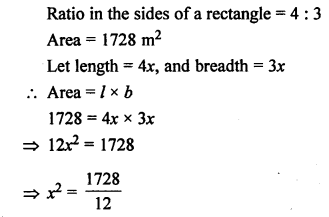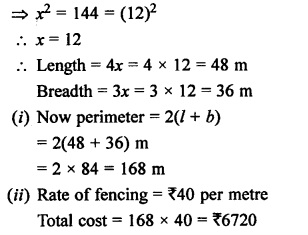Question 4.
A floor is 40 m long and 15 m broad. It is covered with tiles, each measuring 60 cm by 50 cm. Find the number of tiles required to cover the floor.
Solution:Question 5.
The length and breadth of a rectangular piece of land are in the ratio 5 : 3. If the total cost of fencing it at the rate of ₹24 per meter is ₹9600, find its :
(ii) area
(iii) cost of levelling at the rate of ₹60 per m2.
Solution: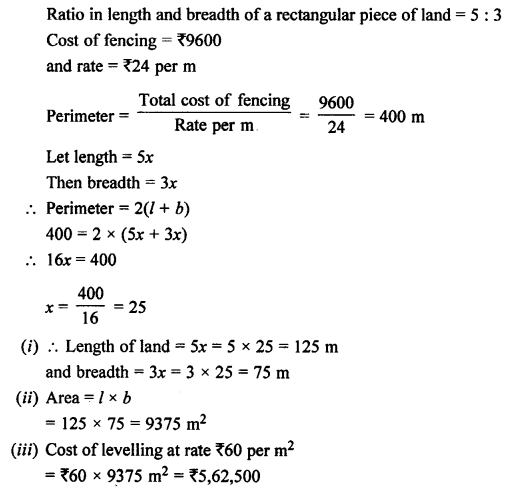Question 6.
Find the area of the square whose perimeter is 56 cm.
Solution:Question 7.
A square lawn is surrounded by a path 2.5 m wide. If the area of the path is 165 m2 find the area of the lawn.
Solution: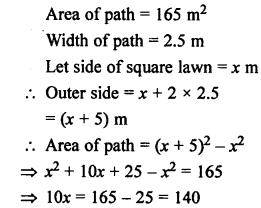Question 8.
For each figure, given below, find the area of shaded region : (All measurements are in cm)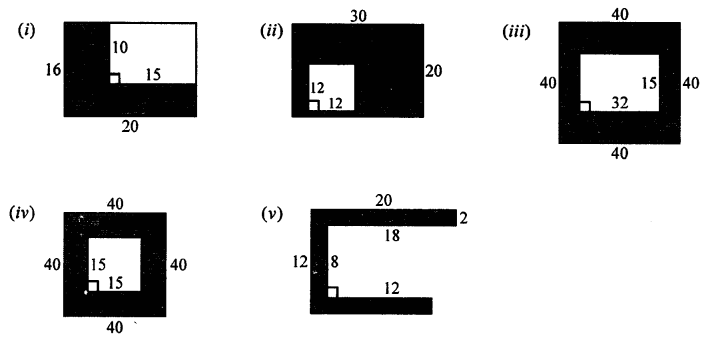Solution: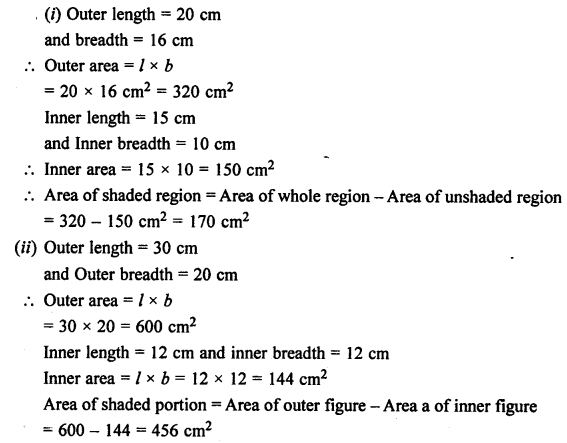Question 9.
One side of a parallelogram is 20 cm and its distance from the opposite side is 16 cm. Find the area of the parallelogram.
Solution:Question 10.
The base of a parallelogram is thrice it height. If its area is 768 cm2, find the base and the height of the parallelogram.
Solution: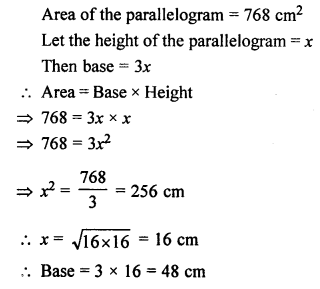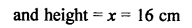Question 11.
Find the area of the rhombus, if its diagonals are 30 cm and 24 cm.
Solution:Question 12.
If the area of a rhombus is 112 cm2 and one of its diagonals is 14 cm, find its other diagonal.
Solution: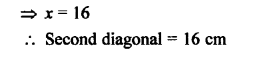Question 13.
One side of a parallelogram is 18 cm and its area is 153 cm2. Find the distance of the given side from its opposite side.
Solution:Question 14.
The adjacent sides of a parallelogram are 15 cm and 10 cm. If the distance between the longer sides is 6 cm, find the distance between the shorter sides.
Solution: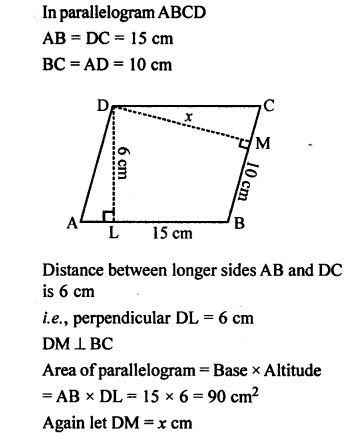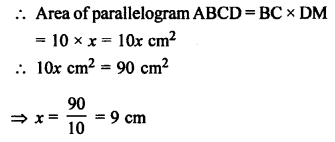Question 15.
The area of a rhombus is 84 cm2 and its perimeter is 56 cm. Find its height.
Solution: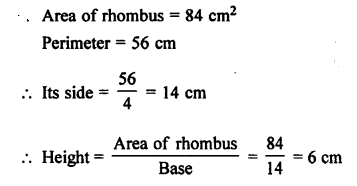Question 16.
Find the area of a triangle whose base is 30 cm and height is 18 cm.
Solution:Question 17.
Find the height of a triangle whose base is 18 cm and area is 270 cm2.
Solution: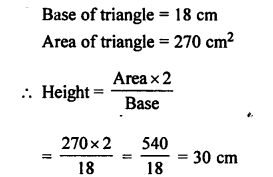Question 18.
The area of a right-angled triangle is 160 cm2. If its one leg is 16 cm long, find the length of the other leg.
Solution: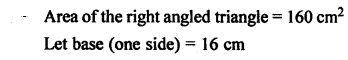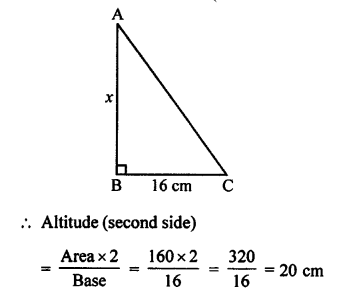Question 19.
Find the area of a right-angled triangle whose hypotenuse is 13 cm long and one of its legs is 12 cm long.
Solution: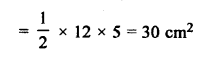Question 20.
Find the area of an equilateral triangle whose each side is 16 cm. (Take $$\sqrt { 3 }$$= 1.73)
Solution:Question 21.
The sides of a triangle are 21 cm, 17 cm and 10 cm. Find its area.
Solution: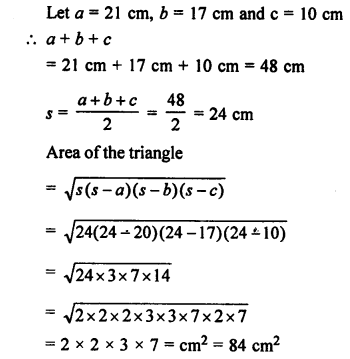Question 22.
Find the area of an isosceles triangle whose base is 16 cm and length of each of the equal sides is 10 cm.
Solution:Question 23.
Find the base of a triangle whose area is 360 cm2and height is 24 cm.
Solution: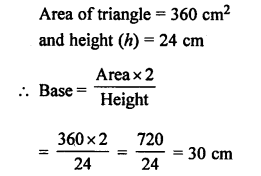Question 24.
The legs of a right-angled triangle are in the ratio 4 :3 and its area is 4056 cm2. Find the length of its legs.
Solution: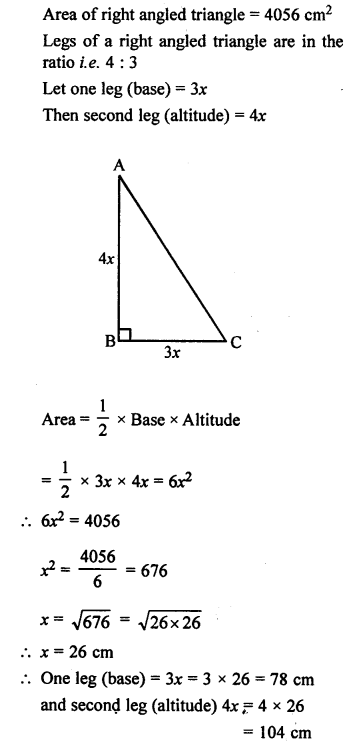Question 25.
The area of an equilateral triangle is (64 x $$\sqrt { 3 }$$ ) cm2– Find the length of each side of the triangle.
Solution:Question 26.
The sides of a triangle are in the ratio 15 : 13 : 14 and its perimeter is 168 cm. Find the area of the triangle.
Solution: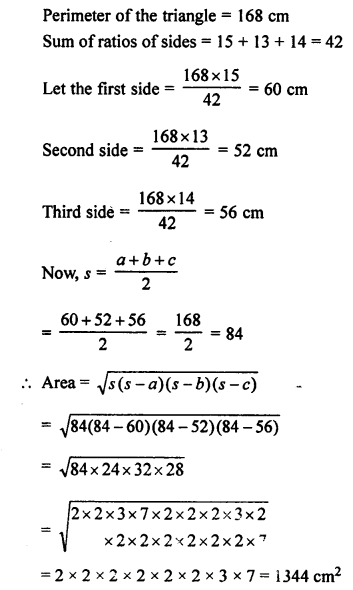Question 27.
The diameter of a circle is 20 cm. Taking π = 3.14, find the circumference and its area.
Solution: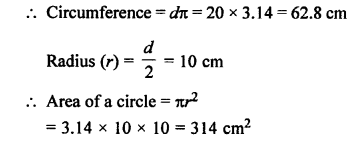Question 28.
The circumference of a circle exceeds its diameter by 18 cm. Find the radius of the circle.
Solution: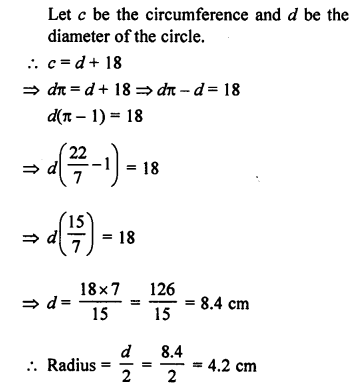Question 29.
The ratio between the radii of two circles is 5 : 7. Find the ratio between their :
(i) circumference
(ii) areas
Solution: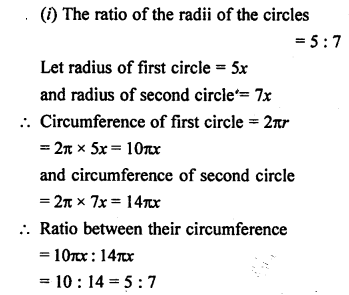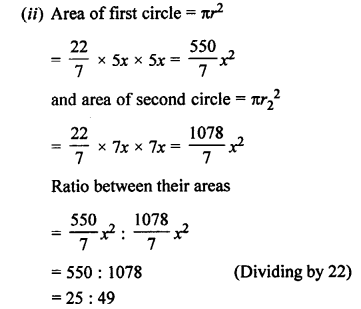Question 30.
The ratio between the areas of two circles is 16 : 9. Find the ratio between their :
(ii) diameters
(iii) circumference
Solution: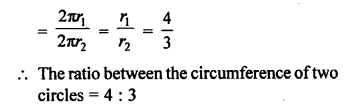Question 31.
A circular racing track has inner circumference 528 m and outer circumference 616 m. Find the width of the track.
Solution: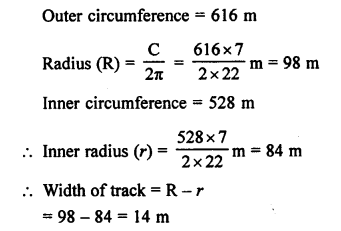Question 32.
The inner circumference of a circular track is 264 m and the width of the track is 7 m. Find:
(i) the radius of the inner track.
(ii) the radius of the outer circumference.
(iii) the length of the outer circumference.
(iv) the cost of fencing the outer circumference at the rate of ₹50 per m.
Solution: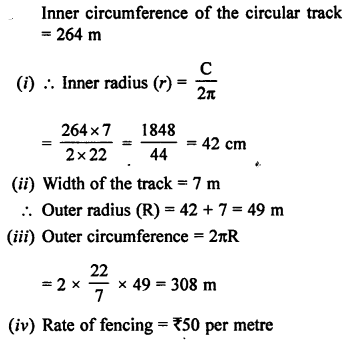Question 33.
The diameter of every wheel of a car is 63 cm. How much distance will the car move during 2000 revolutions of its wheel.
Solution: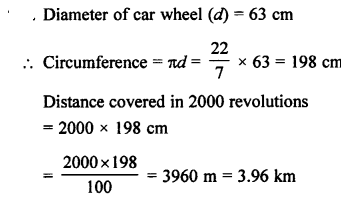Question 34.
The diameter of the wheel of a car is 70 cm. How many revolutions will it make to travel one kilometre?
Solution:Question 35.
A metal wire, when bent in the form of a square of largest area, encloses an area of 484 cm2. Find the length of the wire. If the same wire is bent to a largest circle, find:
(i) radius of the circle formed.
(ii) area of the circle.
Solution: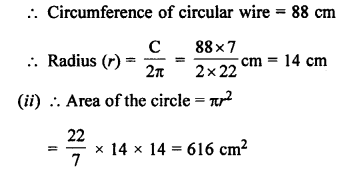Question 36.
A wire is along the boundary of a circle with radius 28 cm. If the same wire is bent in the form of a square, find the area of the square formed.
Solution:Question 37.
The length and the breadth of a rectangular paper are 35 cm and 22 cm. Find the area of the largest circle which can be cut out of this paper.
Solution:Question 38.
From each comer of a rectangular paper (30 cm x 20 cm) a quadrant of a circle of radius 7 cm is cut. Find the area of the remaining paper i.e., shaded portion.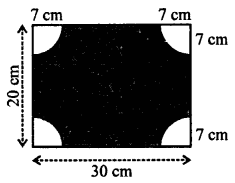Solution: